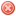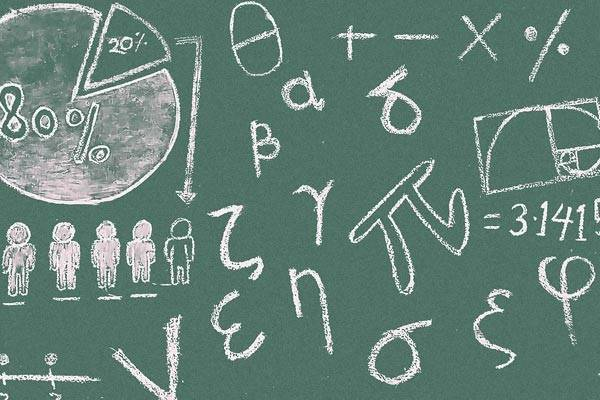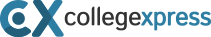# 10 GRE Math Concepts You Need to KnowGRE Quant focuses on a number of clearly defined math concepts. If you know these concepts well, you’ll be able to get a top score in the Quant section. Below are 10 GRE math concepts that are core to GRE success. To familiarize yourself with each of the following concepts, check out our list of GRE books to help you attain your dream GRE Quant score.

## Concept 1: Mental math

In many academic math classes, you’re required to show all of your work, writing out every step. In some advanced classes, you may also need to complete a detailed series of calculations on a graphing calculator, moving step by step to your final calculation. But this really isn’t how math problem-solving works on the GRE. For this exam time is of the essence, so going through every step can actually work against you. Furthermore, “showing your work” is not required at all on the GRE; only your answer matters when your test is scored.

Whenever possible, you should avoid writing out or keying the steps in a math problem. If you see, for example, an equation like 5x + 2 = 1, you could solve for x step-by-step on paper (or with a calculator), as follows:

• 5x + 2 = 1
• 5x + 2 – 2 = 1 – 2
• 5x = 1 – 2
• 5x = -1
• 5x/5 = -1/5
• x = -1/5

But you could probably also get to the result of x = -1/5 completely in your head. The first four steps are really just simple subtraction, and the last two steps are simple division. The trick here is to break the habit of “showing your work.” Always look for chances to do a math operation 100% mentally, as the GRE is full of such opportunities.

## Concept #2: Shortcuts

Think of math shortcuts as the conceptual cousin to mental math. The concept of shortcuts takes mental math a step further. When you use math shortcuts, you don’t just skip writing down a step in a problem; you actually skip doing the step entirely.

Let me give you an example. On the GRE, you might need to find the mean and median of a set of numbers like this one: {13, 17, 21, 25, 29, 33}. Now, for some number sets, the mean and median have to be calculated separately. But you don’t need to calculate these two values separately in a number set where the numbers are spaced evenly (in sequence, each number here is 4 more than the previous one).

In an evenly spaced number sequence like the one above, the mean and median will be the same number. So there’s a shortcut; all you have to do is calculate one of these two values, and you’ll have both mean and median. A median calculation is the fastest possible shortcut here, because you only need the average of the two middle numbers. The average of 21 and 25 is 23, and with that simple “shortcut” operation, you’ve gotten both mean and median very quickly.

There are a wide variety of shortcuts you can use in GRE Math. The concept of GRE shortcuts is so important that you can find plenty of tutorials for different GRE Quant time-savers online. There’s even an entire Facebook group just for GRE Math shortcuts

## Concept #3: Estimation

Here we have yet another conceptual cousin. Estimation is a skill similar to mental math and shortcuts, and it can be a real time saver. Let’s say that the final answer to a multiple choice GRE Quant question is the square root of 12.61 plus the square root of 26. Doing these exact squares and then adding them would be cumbersome. But you can just estimate: 12.61 is a little past halfway between 3 squared (9) and 4 squared (16). So its square root will be just above 3.5, halfway between 3 and 4. And 26 is just a little bit higher than 5 squared (25), so its square root will be just a little more than 5. From there, just look for an answer choice that’s slightly higher than 8.5, the sum of 3.5 and 5.

## Concept # 4: Multiple-choice strategy

If mental math, shortcuts, and estimation are cousin concepts, then multiple-choice strategy is the grandparent of these three cousins. The multiple-choice nature of the GRE Quant section is the reason that mental math, shortcuts, and estimation exist as important GRE math concepts. In GRE Quant, multiple-choice strategy is all about quickly recognizing the properties of numbers and the relationship between numbers. Making these fast connections helps you eliminate the wrong answer choices and get to the right ones efficiently. Always approach GRE Quant multiple-choice questions with a three-prong strategy of mental math, shortcuts, and estimation.

## Concept # 5: Data interpretation

The GRE also requires you to carefully analyze numbers from the data found in graphs, charts, tables, and narrative descriptions. This concept is not unique to the GRE, but it is a concept you may not have dealt with recently, especially if you’ve been out of school for a while. For a top GRE Quant score, make sure you get in some good practice with informational graphics and written descriptions of data.

## Concept # 6: Statistics

Statistics is an important GRE math concept rooted in data interpretation. In GRE Quant, you will often be asked to calculate statistical figures based on the data you are interpreting. You’ll need to understand statistical principles such as percentages and averages and be comfortable with statistics vocabulary, especially “mean,” “median,” and “mode.”

## Concept # 7: Probability

Probability is another conceptual offshoot from data interpretation. Probability measures the likeliness that an event will happen. Probability calculations involve some very specific formulas and math operations. The finer points of probability are too complex to delve into in this article. Fortunately, because probability is so important on the GRE, there are plenty of online resources to help you master this concept. Magoosh’s example GRE probability questions is a good place to start.

## Concept # 8: Arithmetic

“Arithmetic” has a sound of simplicity to a lot of GRE test-takers. It sounds like the basic, pre-algebra math you might have learned in elementary and middle school, before shifting your focus to more sophisticated types of math. In reality, the concept of arithmetic extends far beyond grade school math, and many graduate-level math problems in GRE Quant revolve around arithmetic. On the official GRE website, ETS lists factorization, remainders, exponents, roots, and a number of other important arithmetic components you can expect to find in math problems on the exam.

## Concept # 9: Algebra

This is a GRE math concept you should be pretty familiar with. You started to learn algebra in middle school, and you kept studying this kind of math in high school. You likely took at least one or two algebra courses as an undergrad, possibly more depending on your major. On the GRE, you’ll need to pull all of this past knowledge together and take on some new aspects of algebra that you may not be very familiar with. Alongside its description of GRE arithmetic, the Quant section overview on the official GRE website also lists important aspects of algebra.

## Concept #10: Geometry

Geometry is another GRE math concept that you probably have a lot of past exposure to. As with algebra, test-takers need to combine years of prior learning in geometry as they prepare for GRE geometry. Make sure that you memorize the various GRE geometry formulas you need. Also make sure that you understand the principles behind these formulas. Test them out, practice using them, and get a feel for how the formulas work and what you can accomplish with them.

Test-takers, past and future: what’s your experience like with these GRE math concepts? Did we miss anything? Let us know in the comments.

Note: Did you know you could win a \$10,000 scholarship for college or grad school just by registering on CollegeXpress? This is one of the quickest, easiest scholarships you’ll ever apply for. Register Now »\$10,000

## Are you our next winner?

Register now for our scholarship giveaway

Moultrie, GA

Tampa, FL

New Haven, CT

Tampa, FL